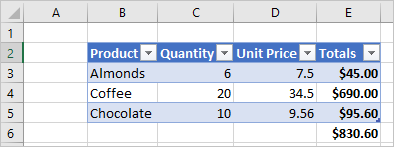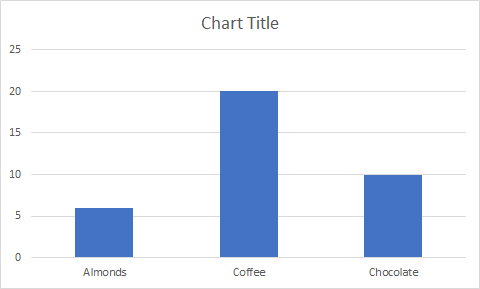# [Excel] Office Scripts

jen·2021년 7월 27일
0

## Office Scripts

목록 보기
1/1

### Create a table

function main(workbook: ExcelScript.Workbook) {
// Get the active worksheet.
let sheet = workbook.getActiveWorksheet();

// Add a table that has headers using the data from B2:E5.
}### Create a chart

function main(workbook: ExcelScript.Workbook) {
// Get the active worksheet.
let sheet = workbook.getActiveWorksheet();

// Create a column chart using the data from B3:C5.
ExcelScript.ChartType.columnStacked,
sheet.getRange("B3:C5")
);

// Set the margin of the chart to be 100 pixels from the top of the screen.
chart.setTop(100);
}## Samples

### Read and log one cell

This sample reads the value of A1 and prints it to the console.

function main(workbook: ExcelScript.Workbook) {
// Get the current worksheet.
let selectedSheet = workbook.getActiveWorksheet();

// Get the value of cell A1.
let range = selectedSheet.getRange("A1");

// Print the value of A1.
console.log(range.getValue());
}

This script logs the value of the current active cell. If multiple cells are selected, the top-leftmost cell will be logged.

function main(workbook: ExcelScript.Workbook) {
// Get the current active cell in the workbook.
let cell = workbook.getActiveCell();

// Log that cell's value.
console.log(The current cell's value is ${cell.getValue()}); } ### ... function main(workbook: ExcelScript.Workbook) { // Get the active worksheet. let sheet = workbook.getActiveWorksheet(); let range = selectedSheet.getUsedRange(true); // If the used range is empty, end the script. if (!range) { console.log(No data on this sheet.); return; } // Log the address of the used range. ex) Sheet1!B2:E5 console.log(Used range for the worksheet:${range.getAddress()});

// 마지막 셀
selectedSheet.getRange(A1:\${range.getCell(7, lastColNumber).getAddress()}).delete(ExcelScript.DeleteShiftDirection.up)
}